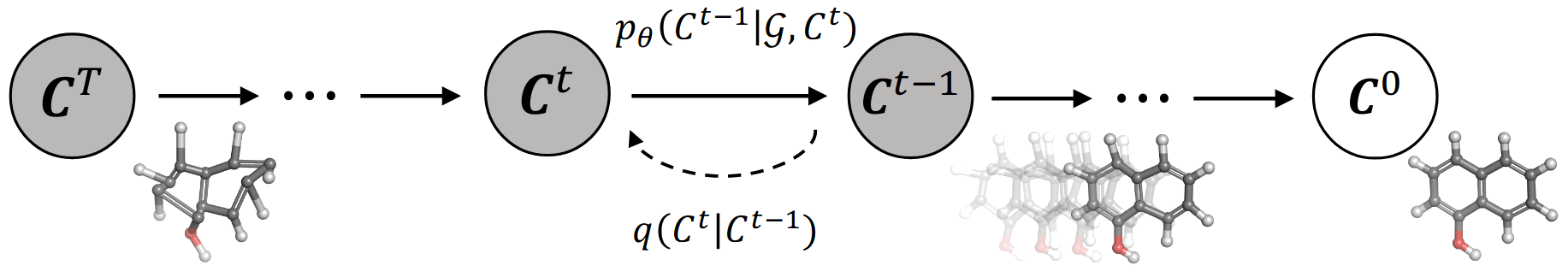GeoDiff: A Geometric Diffusion Model for Molecular Conformation Generation

Diffusion models are used for predicting the stable 3d conformations of a molecule from its 2d graph.

The problem of predicting molecular 3d structure is of fundamental importance in computational chemistry and drug discovery. Given the 2d graph of a molecule, the goal is to predict all of its stable conformations, i.e. all the stable 3d positions of every atom at a given temperature. In recent years, significant progress has been achieved with deep generative models, and even more recently through the use of diffusion models.

Inspired by the diffusion processes in classical non-equilibrium thermodynamics, where heated particles diffuse from original states to a noisy distribution, diffusion models train a neural network to reverse this process and to generate patterns from noisy configurations. Given their natural physical formulation, they are a perfect fit for molecular 3d reconstruction.Figure 1. [Xu22G] Illustration of the diffusion and reverse process of GEODIFF. For diffusion process, noise from fixed posterior distributions $q(\mathcal{C}^t|\mathcal{C}^{t−1})$ is gradually added until the conformation is destroyed. Symmetrically, for generative process, an initial state CT is sampled from standard Gaussian distribution, and the conformation is progressively refined via the Markov kernels $p_\theta(\mathcal{C}^{t−1}|\mathcal{G}, \mathcal{C}^t)$

[Xu22G] describes one implementation of diffusion models for molecular 3d reconstruction. Given the full molecule conformation C, the authors progressively add noise until the 3d structure is lost and only the 2d structure remains. Then, a neural network is trained to re-build the structures at times $t$ from $0$ to $T$. Since physical laws at the atomic level are roto-translational invariant, the diffusion uses Markov kernels with the same invariances.

The new method, called GEODIFF (available on github) is superior or comparable to existing state-of-the-art approaches, especially on larger molecules, which are typically the most challenging.